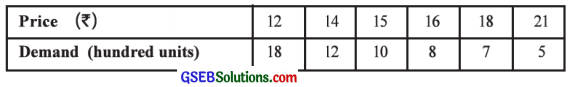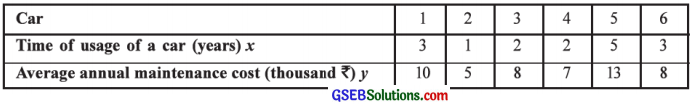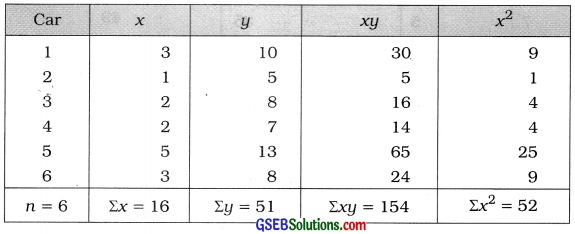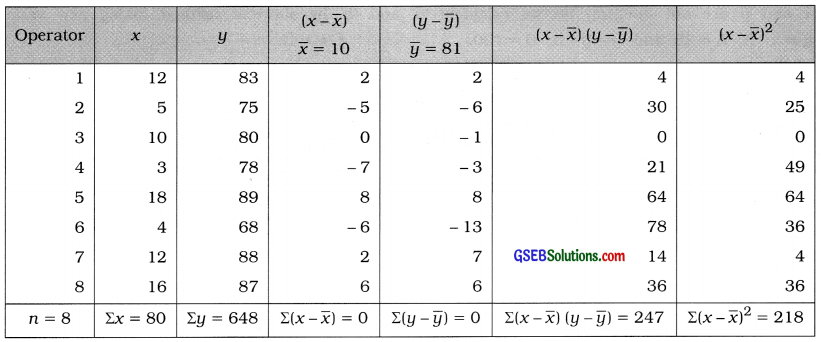# GSEB Solutions Class 12 Statistics Part 1 Chapter 3 Linear Regression Ex 3.1

Gujarat Board Statistics Class 12 GSEB Solutions Part 1 Chapter 3 Linear Regression Ex 3.1 Textbook Exercise Questions and Answers.

## Gujarat Board Textbook Solutions Class 12 Statistics Part 1 Chapter 3 Linear Regression Ex 3.1

Question 1.
From the following data of price (in ₹) and demand (in hundred units) of a commodity, obtain the regression line of demand on price. Also estimate the demand when price is ₹ 20.Here, n = 6; X = Price and Y = Demand
Now, x̄ = $$\frac{\Sigma x}{n}$$ = $$\frac{96}{6}$$ = ₹ 16, ȳ = $$\frac{\Sigma y}{n}$$ = $$\frac{60}{6}$$ = 10 (’00) units.
To obtain the equation of regression line of Y on X,
ŷ = a + bx, we calculate a and b.
x̄ and ȳ are integers. So the table of calculation is prepared as follows:b = $$\frac{\Sigma(x-\bar{x})(y-\bar{y})}{\Sigma(x-\bar{x})^{2}}$$
Putting, Σ(x – x̄) (y – ȳ) = – 67 and Σ(x – x̄)2 = 50 in the formula,
b = $$\frac{-67}{50}$$
= – 1.34

a = ȳ – bx̄
Putting ȳ = 10; x̄ = 16 and b = – 1.34, we get
a = 10 – (- 1.34) (16)
= 10 + 21.44
= 31.44

Regression line of demand (Y) on price (X) :
Putting a = 31.44 and b = – 1.34 in ŷ = a + bx, we get
ŷ = 31.44 – 1.34x

When price X = ₹ 20, the estimate of demand Y:
Putting x = 20 in ŷ = 31.44 – 1.34x, we get
ŷ = 31.44 – 1.34 (20)
= 31.44 – 26.8 = 4.64
Hence, when price is ₹ 20, the estimated demand Y obtained is ŷ = 4.64 (’00) units.Question 2.
To study the relationship between the time of usage of cars and its average annual maintenance cost, the following information is obtained:Obtain the regression line of Y on X. Find an estimate of average annual maintenance cost when the usage time of a car is 5 years. Also find its error.
Here, n = 6; X = Time of usage of a car and Y = Annual average maintenance cost
Now, x̄ = $$\frac{\Sigma x}{n}$$ = $$\frac{16}{6}$$ = 2.67; ȳ = $$\frac{\Sigma y}{n}$$ = $$\frac{51}{6}$$ = 8.5
We obtain the regression line of average annual maintenance cost (Y) on the time of usage (X) ŷ = a + bx.

The values of X and Y are not large. So we calculate the values of a and b by preparing the following table:b = $$\frac{n \Sigma x y-(\Sigma x)(\Sigma y)}{n \Sigma x^{2}-(\Sigma x)^{2}}$$
Putting n = 6, Σxy = 154; Σx = 16; Σy = 15 and Σx2 = 52 in the formula
= $$\frac{6(154)-(16)(51)}{6(52)-(16)^{2}}$$
= $$\frac{924-816}{312-256}$$
= $$\frac{108}{56}$$ = 1.93

a = ȳ – bx̄
Putting ȳ = 8.5; x̄ = 2.67 and b = 1.93, we get,
a = 8.5 – 1.93 (2.67)
= 8.5 – 5.15
= 3.35

Regression line of Y on X:
Putting a = 3.35 and b = 1.93 in ŷ = a + bx, we obtain ŷ = 3.35 + 1.93x

The estimate of average annual maintenance cost Y when usage of car X = 5 years:
Putting x = 5 in ŷ = 3.35 + 1.93x, we get
ŷ = 3.35 + 1.93 (5)
= 3.35 + 9.65 = ₹ 13 thousand
Hence, the estimate of average annual maintenance cost obtained is y = ₹ 13 thousand.

Error: ŷ = 13, y = 13
∴ Error = ŷ – y = 13 – 13 = 0
Hence, point (5, 13) is on he fitted regression line ŷ = 3.35 + 1.93 x.Question 3.
The information for a year regarding the average rainfall (in cm) and total production of crop (in tons) of five districts is given below:Find the regression line of production of crop on rainfall and estimate the crop if average rainfall is 35 cm.
Here, n = 5; X = Average rainfall and Y = Crop
Now, x̄ = $$\frac{\Sigma x}{n}$$ = $$\frac{155}{5}$$ = 31 cm; ȳ = $$\frac{\Sigma y}{n}$$ = $$\frac{450}{5}$$ = 90 tons
We obtain the regression line of crop (Y) on the average rainfall (X) ŷ = a + bx.
x̄ and ȳ are integers. So to calculate a and b, we prepare the following table:b = $$\frac{\Sigma(x-\bar{x})(y-\bar{y})}{\Sigma(x-\bar{x})^{2}}$$
Putting, Σ(x – x̄) (y – ȳ) = 75 and Σ(x – x̄)2 = 90 in the formula,
b = $$\frac{75}{50}$$
= 0.83

a = ȳ – bx̄
Putting ȳ = 90; x̄ = 31 and b = 0.83, we get
a = 90 – 0.83 (31)
= 90 – 25.73
= 64.27

Regression line of crop (Y) on average rainfall (X) :
Putting b = 0.83 and a = 64.27 in ŷ = a + bx, we get
ŷ = 64.27 + 0.83x.

Estimate of Y when X = 35 cm :
Putting x = 35 in ŷ = 64.27 + 0.83x, we get
ŷ = 64.27 + 0.83 (35) = 64.27 + 29.05 = 93.32
Hence, the estimate of crop obtained is ŷ = 93.32 tons.Question 4.
The following data gives the experience of machine operators and their performance ratings:Calculate the regression line of performance ratings on the experience and estimate the performance rating of an operator having 7 years of experience.
Here, n = 8; X = Experience and Y = Performance rating.
Now, x̄ = $$\frac{\Sigma x}{n}$$ = $$\frac{80}{8}$$ = 10 year; ȳ = $$\frac{\Sigma y}{n}$$ = $$\frac{648}{8}$$ = 81
We obtain the regression line of Performance rating (Y) on experience (X) ŷ = a + bx.
x̄ and ȳ are integers. So to calculate a and b we prepare the following table:b = $$\frac{\Sigma(x-\bar{x})(y-\bar{y})}{\Sigma(x-\bar{x})^{2}}$$
Putting, Σ(x – x̄) (y – ȳ) = 247 and Σ(x – x̄)2 = 218 in the formula,
b = $$\frac{247}{218}$$
= 1.13

a = ȳ – bx̄
Putting ȳ = 81; x̄ = 10 and b = 1.13, we get
a = 81 – 1.13 (31)
= 81 – 11.3
= 69.7

Regression line of Y on X:
Putting a = 69.7 and b = 1.13 in ŷ = a + bx, we get
ŷ = 69.7 + 1.13x

Estimate of Y when X = 7 years :
Putting x = 7 in ŷ = 69.7 + 1.13x, we get
ŷ = 69.7 + 1.13 (7)
= 69.7 + 7.91
= 77.61
Hence, the estimate of performance rating obtained is ŷ = 77.61.